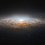# A Family Of Puzzling Infinite Continued Fractions

We can easily figure out that

$\large 1+\frac{1}{1+\frac{1}{1+\frac{1}{1+\frac{1}{1+\frac{1}{1+\frac{1}{1+\ddots}}}}}}=\phi$

where $\phi$ is the 'golden ratio' $1.6180339...$.

(Proof: $1+\frac{1}{1+\frac{1}{1+\frac{1}{1+\frac{1}{1+\frac{1}{1+\frac{1}{1+\ddots}}}}}}$ is equal to $1$ plus its reciprocal, or $x=1+\frac{1}{x}$. After multiplying both sides by $x$ and moving all of the terms to the left, we obtain

$x^2-x-1=0$

which can be evaluated to give the solution $x=\frac{1\pm\sqrt5}{2}$.)

One can see that a continued fraction of this form can be generalized for any continued fraction of the form

$n+\frac{m}{n+\frac{m}{n+\frac{m}{n+\frac{m}{n+\frac{m}{n+\frac{m}{n+\ddots}}}}}}$

which has a solution $\frac{n\pm\sqrt{n^2+4m}}{2}$. I will omit the derivation, which is easy to derive by modifying the proof above.

$\large 1+\frac{1}{2+\frac{2}{3+\frac{3}{4+\frac{4}{5+\frac{5}{6+\frac{6}{7+\frac{7}{\ddots(n-1)+\frac{n-1}{n}\ddots}}}}}}}$

What is the limit of this continued fraction as $n\to\infty$? What is the limit as $n\to\infty$ of any continued fraction of the form

$\large m+\frac{m}{(m+1)\frac{m+1}{(m+2)+\frac{m+2}{(m+3)+\frac{m+3}{(m+4)+\frac{m+4}{(m+5)+\frac{m+6}{(m+7)+\frac{m+7}{\ddots(m+n-1)+\frac{m+n-1}{\ddots}}}}}}}}$Note by Andrei Li
2 years, 12 months ago

This discussion board is a place to discuss our Daily Challenges and the math and science related to those challenges. Explanations are more than just a solution — they should explain the steps and thinking strategies that you used to obtain the solution. Comments should further the discussion of math and science.

When posting on Brilliant:

• Use the emojis to react to an explanation, whether you're congratulating a job well done , or just really confused .
• Ask specific questions about the challenge or the steps in somebody's explanation. Well-posed questions can add a lot to the discussion, but posting "I don't understand!" doesn't help anyone.
• Try to contribute something new to the discussion, whether it is an extension, generalization or other idea related to the challenge.

MarkdownAppears as
*italics* or _italics_ italics
**bold** or __bold__ bold
- bulleted- list
• bulleted
• list
1. numbered2. list
1. numbered
2. list
Note: you must add a full line of space before and after lists for them to show up correctly
paragraph 1paragraph 2

paragraph 1

paragraph 2

[example link](https://brilliant.org)example link
> This is a quote
This is a quote
    # I indented these lines
# 4 spaces, and now they show
# up as a code block.

print "hello world"
# I indented these lines
# 4 spaces, and now they show
# up as a code block.

print "hello world"
MathAppears as
Remember to wrap math in $$ ... $$ or $ ... $ to ensure proper formatting.
2 \times 3 $2 \times 3$
2^{34} $2^{34}$
a_{i-1} $a_{i-1}$
\frac{2}{3} $\frac{2}{3}$
\sqrt{2} $\sqrt{2}$
\sum_{i=1}^3 $\sum_{i=1}^3$
\sin \theta $\sin \theta$
\boxed{123} $\boxed{123}$